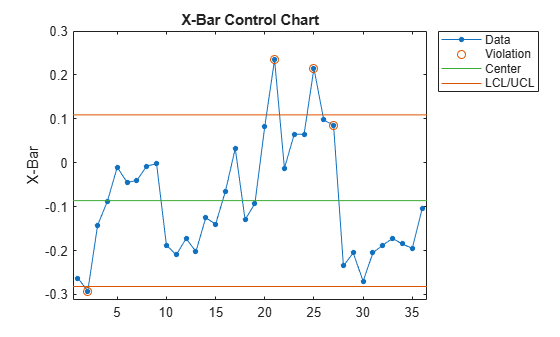controlrules

Western Electric and Nelson control rules

Syntax

R = controlrules('rules',x,cl,se)
[R,RULES] = controlrules('rules',x,cl,se)

Description

R = controlrules('rules',x,cl,se) determines which points in the vector x violate the control rules in rules. cl is a vector of center-line values. se is a vector of standard errors. (Typically, control limits on a control chart are at the values cl3*se and cl + 3*se.) rules is the name of a control rule, or a string array or cell array containing multiple control rule names. If x has n values and rules contains m rules, then R is an n-by-m logical array, with R(i,j) assigned the value 1 if point i violates rule j, 0 if it does not.

The following are accepted values for rules (specified inside single quotes):

• we1 — 1 point above cl + 3*se

• we2 — 2 of 3 above cl + 2*se

• we3 — 4 of 5 above cl + se

• we4 — 8 of 8 above cl

• we5 — 1 below cl 3*se

• we6 — 2 of 3 below cl 2*se

• we7 — 4 of 5 below cl se

• we8 — 8 of 8 below cl

• we9 — 15 of 15 between cl se and cl + se

• we10 — 8 of 8 below cl se or above cl + se

• n1 — 1 point below cl 3*se or above cl + 3*se

• n2 — 9 of 9 on the same side of cl

• n3 — 6 of 6 increasing or decreasing

• n4 — 14 alternating up/down

• n5 — 2 of 3 below cl 2*se or above cl + 2*se, same side

• n6 — 4 of 5 below cl se or above cl + se, same side

• n7 — 15 of 15 between cl se and cl + se

• n8 — 8 of 8 below cl se or above cl + se, either side

• we — All Western Electric rules

• n — All Nelson rules

For multi-point rules, a rule violation at point i indicates that the set of points ending at point i triggered the rule. Point i is considered to have violated the rule only if it is one of the points violating the rule's condition.

Any points with NaN as their x, cl, or se values are not considered to have violated rules, and are not counted in the rules for other points.

Control rules can be specified in the controlchart function as values for the 'rules' parameter.

[R,RULES] = controlrules('rules',x,cl,se) returns a cell array of text RULES listing the rules applied.

Examples

collapse all

Create an Xbar chart using the we2 rule to mark out of control measurements.

st = controlchart(runout,'rules','we2');
x = st.mean;
cl = st.mu;
se = st.sigma./sqrt(st.n);
hold on
plot(cl+2*se,'m')You can see the out of control points marked with a red circle.

Use controlrules to identify the measurements that violate the control rule.

R = controlrules('we2',x,cl,se);
I = find(R)
I = 6×1

21
23
24
25
26
27